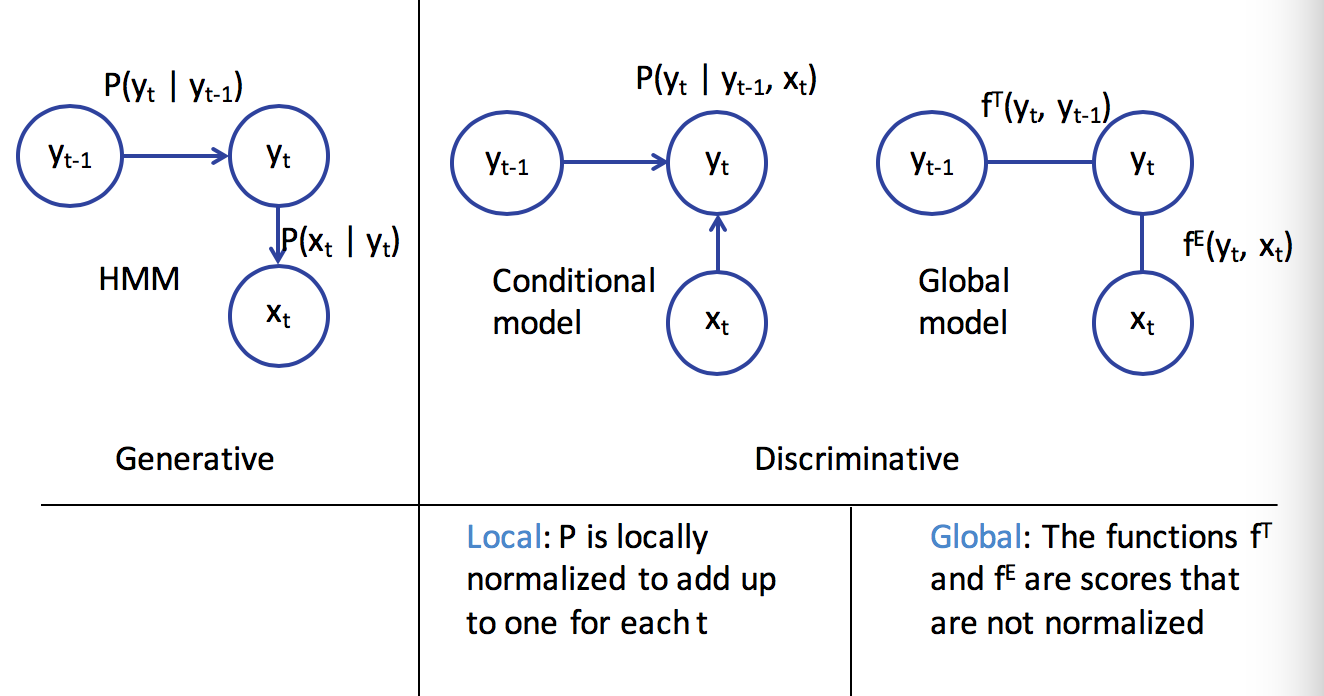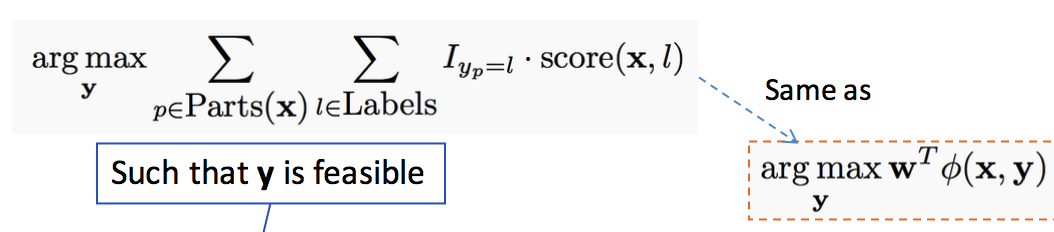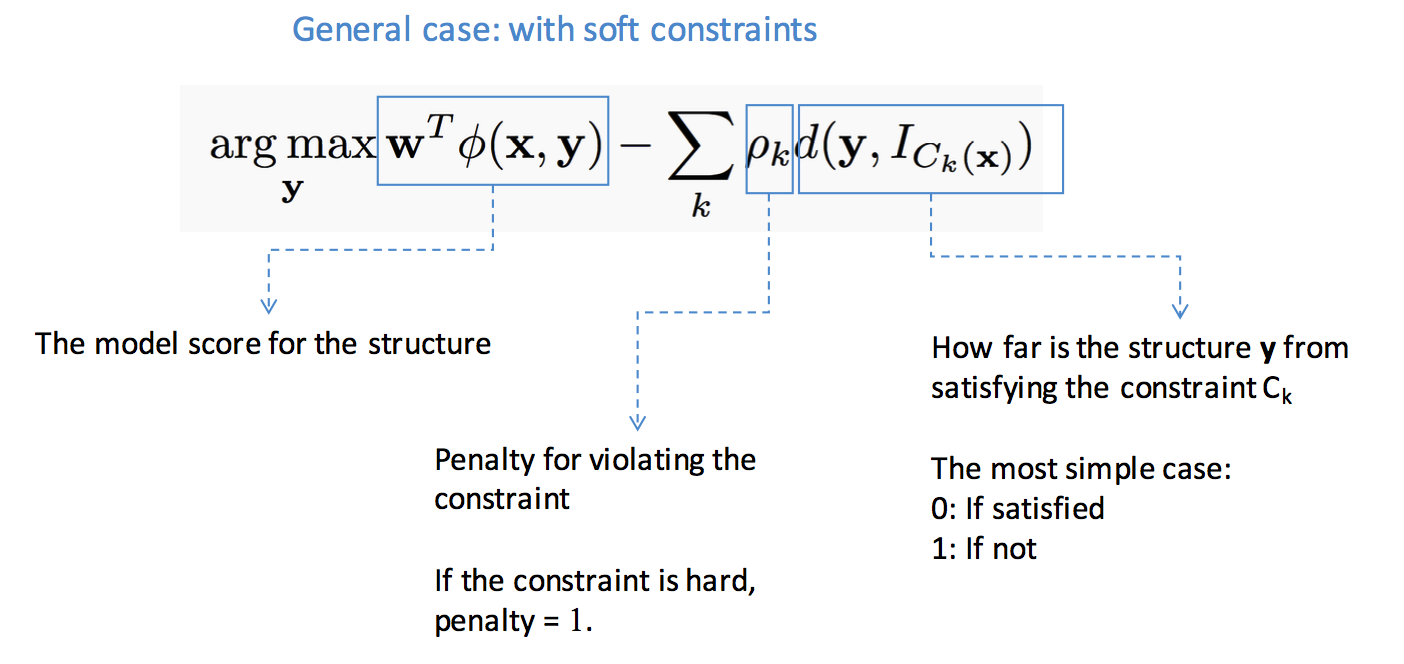structure learning

# introduction

1. structured prediction - have multiple independent output variables
• output assignments are evaluated jointly
• requires joint (global) inference
• can’t use classifier because output space is combinatorially large
• three steps
1. model - pick a model
2. learning = training
3. inference = testing
2. representation learning - picking features
• usually use domain knowledge
3. combinatorial - ex. map words to higher dimensions
4. hierarchical - ex. first layers of CNN

# structure

• structured output can be represented as a graph
• outputs y
• inputs x
• two types of info are useful
• relationships between x and y
• relationships betwen y and y
• complexities
1. modeling - how to model?
2. train - can’t train separate weight vector for each inference outcome
3. inference - can’t enumerate all possible structures
• need to score nodes and edges
• could score nodes and edges independently
• could score each node and its edges together

# sequential models## sequence models

• goal: learn distribution $P(x_1,…,x_n)$ for sequences $x_1,…,x_n$
• ex. text generation
• discrete Markov model
• $P(x_1,…,x_n) = \prod_i P(x_i \vert x_{i-1})$
• requires
1. initial probabilites
2. transition matrix
• mth order Markov model - keeps history of previous m states
• each state is an observation

## conditional models and local classifiers - discriminative model

• conditional models = discriminative models
• goal: model $P(Y\vert X)$
• learns the decision boundary only
• ignores how data is generated (like generative models)
• ex. log-linear models
• $P(\mathbf{y\vert x,w}) = \frac{exp(w^T \phi (x,y))}{\sum_y’ exp(w^T \phi (x,y’))}$
• training: $w = \underset{w}{argmin} \sum log : P(y_i\vert x_i,w)$
• ex. next-state model
• $P(\mathbf{y}\vert \mathbf{x})=\prod_i P(y_i\vert y_{i-1},x_i)$
• ex. maximum entropy markov model
• $P(y_i\vert y_{i-1},x) \propto exp( w^T \phi(x,i,y_i,y_{i-1}))$
• adds more things into the feature representation than HMM via $\phi$
• has label bias problem
• if state has fewer next states they get high probability
• effectively ignores x if $P(y_i\vert y_{i-1})$ is too high
• ex. conditional random fields=CRF
• a global, undirected graphical model
• divide into factors
• $P(Y\vert x) = \frac{1}{Z} \prod_i exp(w^T \phi (x,y_i,y_{i-1}))$
• $Z = \sum_{\hat{y}} \prod_i exp(w^T \phi (x,\hat{y_i},\hat{y}_{i-1}))$
• $\phi (x,y) = \sum_i \phi (x,y_i,y_{i-1})$
• prediction via Viterbi (with sum instead of product)
• training
• maximize log-likelihood $\underset{W}{max} -\frac{\lambda}{2} w^T w + \sum log : P(y_I\vert x_I,w)$
• requires inference
• linear-chain CRF - only looks at current and previous labels
• ex. structured perceptron
• HMM is a linear classifier

# constrained conditional models

## consistency of outputs and the value of inference

• ex. POS tagging - sentence shouldn’t have more than 1 verb
• inference
• a global decision comprising of multiple local decisions and their inter-dependencies
1. local classifiers
2. constraints
• learning

• global - learn with inference (computationally difficult)

## hard constraints and integer programs

•## soft constraints

•# inference

• inference constructs the output given the model
• goal: find highest scoring state sequence

• $argmax_y : score(y) = argmax_y w^T \phi(x,y)$
• naive: score all and pick max - terribly slow
• viterbi - decompose scores over edges
• questions
1. exact v. approximate inference
• exact - search, DP, ILP
• approximate = heuristic - Gibbs sampling, belief propagation, beam search, linear programming relaxations
2. randomized v. deterministic
• if run twice, do you get same answer
• ILP - integer linear programs
• combinatorial problems can be written as integer linear programs
• many commercial solvers and specialized solvers
• NP-hard in general
• special case of linear programming - minimizing/maximizing a linear objective function subject to a finite number of linear constraints (equality or inequality)
• in general, $c = \underset{c}{argmax}: c^Tx$ subject to $Ax \leq b$
• maybe more constraints like $x \geq 0$
• the constraint matrix defines a polytype
• only the vertices or faces of the polytope can be solutions
• $\implies$ can be solved in polynomial time
• in ILP, each $x_i$ is an integer
• LP-relaxation - drop the integer constraints and hope for the best
• 0-1 ILP - $\mathbf{x} \in {0,1}^n$
• decision variables for each label $z_A = 1$ if output=A, 0 otherwise
• don’t solve multiclass classification with an ILP solver (makes it harder)
• belief propagation
• variable elimination
1. fix an ordering of the variables
2. iteratively, find the best value given previous neighbors
• use DP
• ex. Viterbi is max-product variable elimination
• when there are loops, require approximate solution
• uses message passing to determine marginal probabilities of each variable
• message $m_{ij}(x_j)$ high means node i believes $P(x_j)$ is high
• use beam search - keep size-limited priority queue of states

# learning protocols

## structural svm

• $\underset{w}{min} : \frac{1}{2} w^T w + C \sum_i \underset{y}{max} (w^T \phi (x_i,y)+ \Delta(y,y_i) - w^T \phi(x_i,y_i) )$

## empirical risk minimization

• ex. $f(x) = max ( f_1(x), f_2(x))$, solve the max then compute gradient of whichever function is argmax